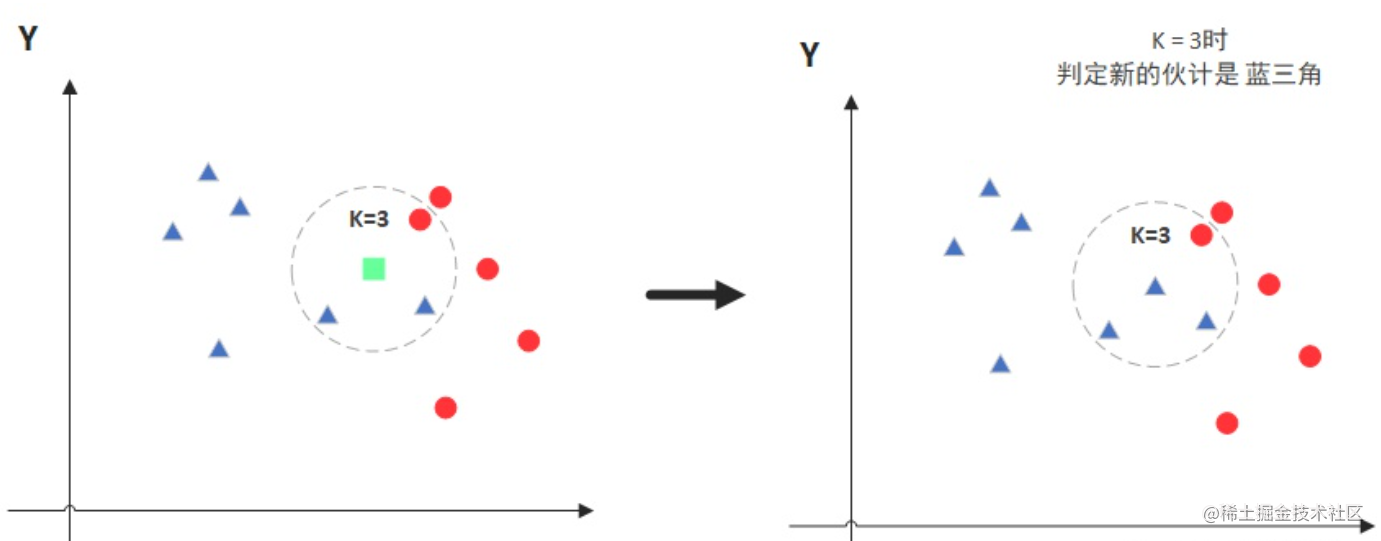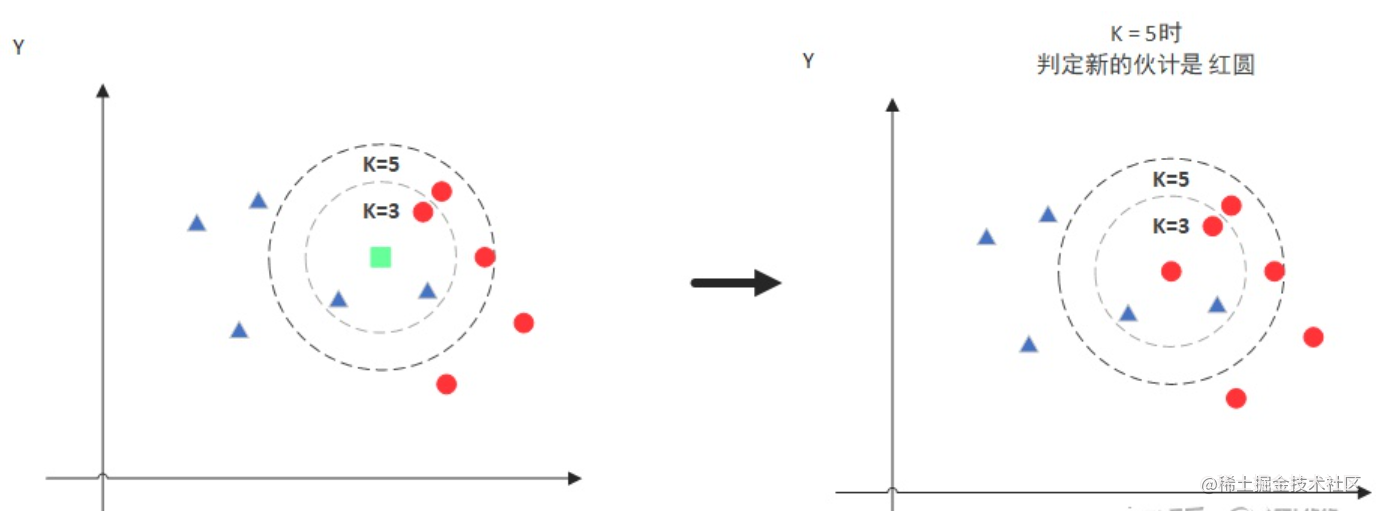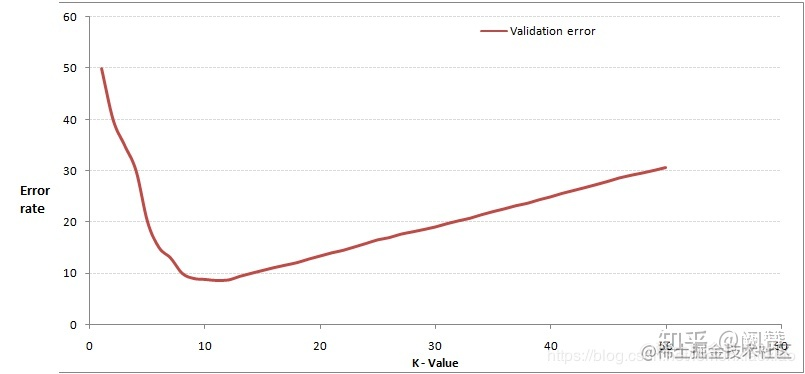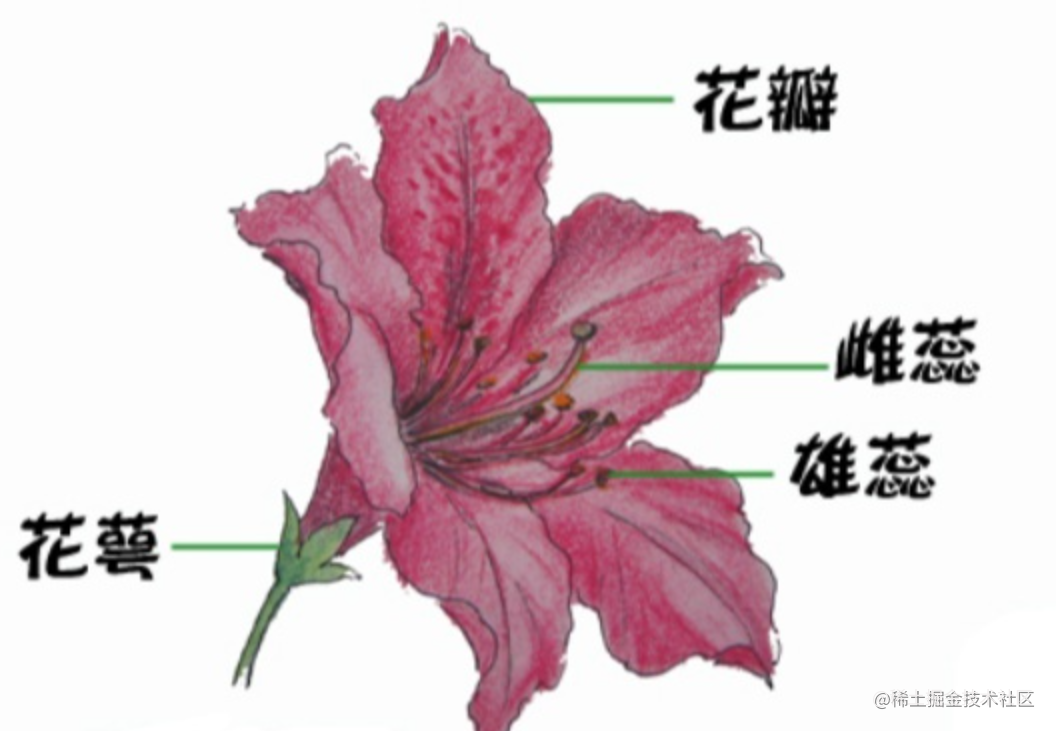# KNN針對的是分類問題

KNN針對分類問題，通過改變決策規則也可用於迴歸問題。

# 定義

K Nearest Neighbor演算法，如果一個樣本在特徵空間中的k個最近似（即特徵空間中最鄰近）的樣本中的大多數屬於某一個類別，則該樣本也屬於這個類別。

注意：KNN 演算法是有監督學習中的分類演算法，注意與K-means演算法區分
K-means為無監督學習演算法，二者有本質區別，一個是分類，一個是聚類。


# 核心思想

KNN 的全稱是 K Nearest Neighbors，意思是 K 個最近的鄰居。 KNN 的原理就是當預測一個新的值 x 的時候，根據它距離最近的 K 個點是什麼類別來判斷 x 屬於哪個類別。## 距離計算

$$d(x, y):=\sqrt{\left(x_{1}-y_{1}\right)^{2}+\left(x_{2}-y_{2}\right)^{2}+\cdots+\left(x_{n}-y_{n}\right)^{2}}=\sqrt{\sum_{i=1}^{n}\left(x_{i}-y_{i}\right)^{2}} .$$ KNN 演算法最簡單粗暴的就是將預測點與所有點距離進行計算，然後儲存並排序，選出前面 K 個值看看哪些類別比較多。但其實也可以通過一些資料結構來輔助，比如最大堆，這裡就不多做介紹，有興趣可以百度最大堆相關資料結構的知識。

## K值選擇

• 對於K值的選擇，一般根據樣本分佈選擇一個較小的值，然後通過交叉驗證來選擇一個比較合適的最終值；
• 當選擇比較小的K值的時候，表示使用較小領域中的樣本進行預測，訓練誤差會減小，但是會導致模型變得複雜，容易導致過擬合；
• 當選擇較大的K值的時候，表示使用較大領域中的樣本進行預測，訓練誤差會增大，同時會使模型變得簡單，容易導致欠擬合；# 演算法實現

## Sklearn KNN引數概述

def KNeighborsClassifier(n_neighbors = 5, weights='uniform', algorithm = '', leaf_size = '30', p = 2, metric = 'minkowski', metric_params = None, n_jobs = None ) 其中：

• n_neighbors：這個值就是指 KNN 中的 “K”了。前面說到過，通過調整 K 值，演算法會有不同的效果。
• weights（權重）：最普遍的 KNN 演算法無論距離如何，權重都一樣，但有時候我們想搞點特殊化，比如距離更近的點讓它更加重要。這時候就需要 weight 這個引數了，這個引數有三個可選引數的值，決定了如何分配權重。引數選項如下：

* ‘uniform’：不管遠近權重都一樣，就是最普通的 KNN 演算法的形式。
* ‘distance’：權重和距離成反比，距離預測目標越近具有越高的權重。
* 自定義函式：自定義一個函式，根據輸入的座標值返回對應的權重，達到自定義權重的目的。

• algorithm：在 Sklearn 中，要構建 KNN 模型有三種構建方式：

1. 暴力法，就是直接計算距離儲存比較的那種方式。
2. 使用 Kd 樹構建 KNN 模型。
3. 使用球樹構建。

其中暴力法適合資料較小的方式，否則效率會比較低。如果資料量比較大一般會選擇用 Kd 樹構建 KNN 模型，而當 Kd 樹也比較慢的時候，則可以試試球樹來構建 KNN。引數選項如下：

* ‘brute’ ：蠻力實現。
* ‘kd_tree’：KD 樹實現 KNN。
* ‘ball_tree’：球樹實現 KNN。
* ‘auto’： 預設引數，自動選擇合適的方法構建模型。


不過當資料較小或比較稀疏時，無論選擇哪個最後都會使用 ‘brute’。

• leaf_size：如果是選擇蠻力實現，那麼這個值是可以忽略的。當使用 Kd 樹或球樹，它就是停止建子樹的葉子節點數量的閾值。預設30，但如果資料量增多這個引數需要增大，否則速度過慢不說，還容易過擬合。

• p：和 metric 結合使用，當 metric 引數是 “minkowski” 的時候，p=1 為曼哈頓距離， p=2 為歐式距離。預設為p=2。
• metric：指定距離度量方法，一般都是使用歐式距離。

* ‘euclidean’ ：歐式距離。
* ‘manhattan’：曼哈頓距離。
* ‘chebyshev’：切比雪夫距離。
* ‘minkowski’： 閔可夫斯基距離，預設引數。

• n_jobs：指定多少個CPU進行運算，預設是-1，也就是全部都算。

## KNN程式碼示例

### Sklearn 鳶尾花from sklearn.datasets import load_iris from sklearn.model_selection import cross_val_score import matplotlib.pyplot as plt from sklearn.neighbors import KNeighborsClassifier

# 讀取鳶尾花資料集

iris = load_iris() x = iris.data y = iris.target k_range = range(1, 31) k_error = []

# 迴圈，取k=1到k=31，檢視誤差效果

for k in k_range: knn = KNeighborsClassifier(n_neighbors=k) #cv引數決定資料集劃分比例，這裡是按照5:1劃分訓練集和測試集 scores = cross_val_score(knn, x, y, cv=6, scoring='accuracy') k_error.append(1 - scores.mean())

# 畫圖，x軸為k值，y值為誤差值

plt.plot(k_range, k_error) plt.xlabel('Value of K for KNN') plt.ylabel('Error') plt.show()  有了這張圖，我們就能明顯看出 K 值取多少的時候誤差最小，這裡明顯是 K=11 最好。當然在實際問題中，如果資料集比較大，那為減少訓練時間，K 的取值範圍可以縮小。

n_neighbors = 11

# 匯入一些要玩的資料 iris = datasets.load_iris() x = iris.data[:, :2] # 我們只採用前兩個feature,方便畫圖在二維平面顯示 y = iris.target

h = .02 # 網格中的步長

# 建立彩色的圖 cmap_light = ListedColormap(['#FFAAAA', '#AAFFAA', '#AAAAFF']) cmap_bold = ListedColormap(['#FF0000', '#00FF00', '#0000FF'])

# weights是KNN模型中的一個引數，上述引數介紹中有介紹，這裡繪製兩種權重引數下KNN的效果圖

for weights in ['uniform', 'distance']: # 建立了一個knn分類器的例項，並擬合數據 clf = neighbors.KNeighborsClassifier(n_neighbors, weights=weights) clf.fit(x, y)

# 繪製決策邊界，為此，我們將為每個分配一個顏色
# 來繪製網格中的點 [x_min, x_max]x[y_min, y_max].
x_min, x_max = x[:, 0].min() - 1, x[:, 0].max() + 1
y_min, y_max = x[:, 1].min() - 1, x[:, 1].max() + 1
xx, yy = np.meshgrid(np.arange(x_min, x_max, h),
np.arange(y_min, y_max, h))
Z = clf.predict(np.c_[xx.ravel(), yy.ravel()])

# 將結果放入一個彩色圖中
Z = Z.reshape(xx.shape)
plt.figure()
plt.pcolormesh(xx, yy, Z, cmap=cmap_light)

# 繪製訓練點
plt.scatter(x[:, 0], x[:, 1], c=y, cmap=cmap_bold)
plt.xlim(xx.min(), xx.max())
plt.ylim(yy.min(), yy.max())
plt.title("3-Class classification (k = %i, weights = '%s')" % (n_neighbors, weights))


plt.show() 

# 演算法特點

KNN是一種非參的、惰性的演算法模型。

# 演算法優缺點

## 優點

1. 簡單易用。相比其他演算法，KNN 算是比較簡潔明瞭的演算法，即使沒有很高的數學基礎也能搞清楚它的原理。
2. 模型訓練時間快，上面說到 KNN 演算法是惰性的，這裡也就不再過多講述。
3. 預測效果好。
4. 對異常值不敏感。

## 缺點

1. 對記憶體要求較高，因為該演算法儲存了所有訓練資料。
2. 預測階段可能很慢。
3. 對不相關的功能和資料規模敏感。
「其他文章」